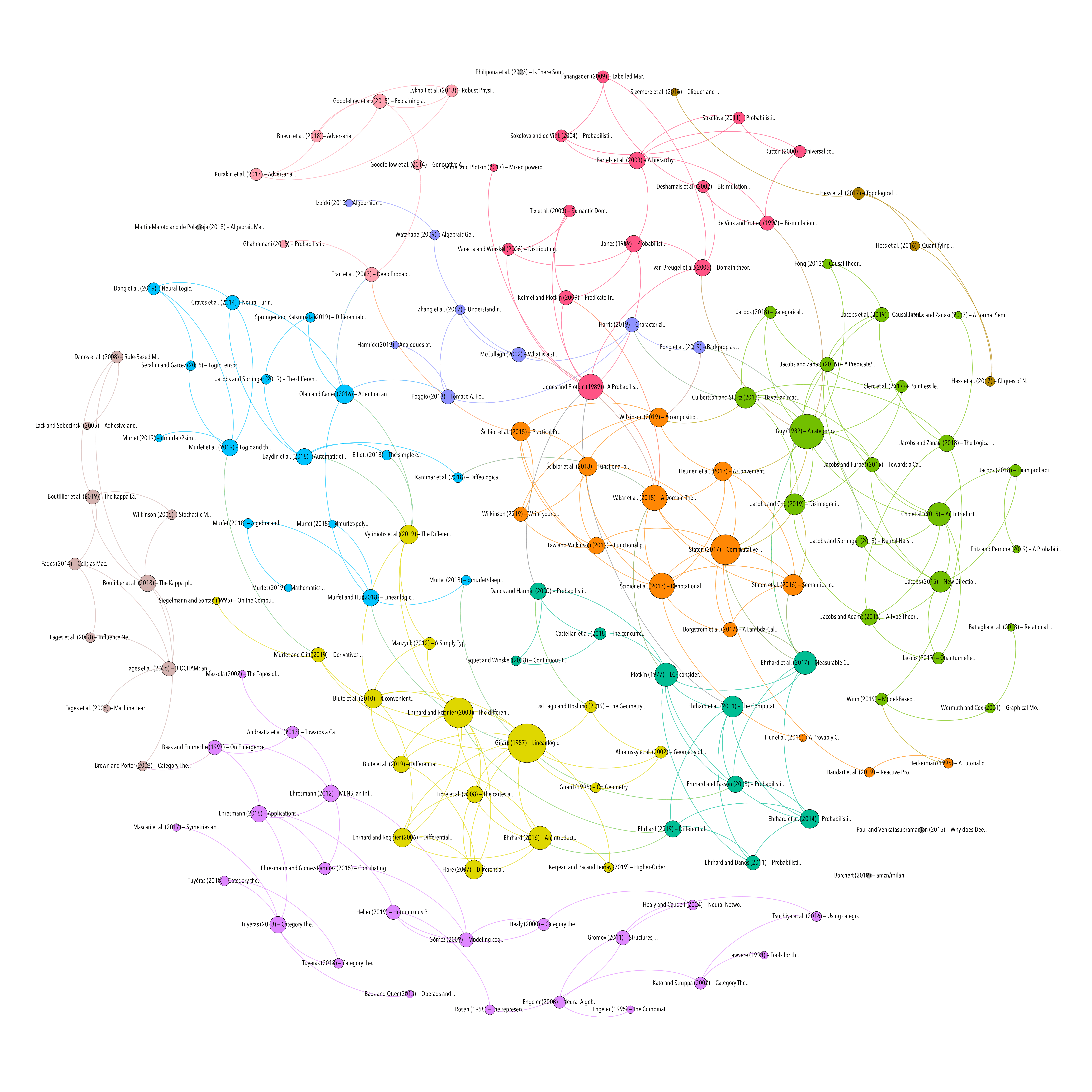MACHINE LEARNING
PROBABILITY & STATISTICS
Publication year

## A Tutorial on Learning With Bayesian Networks

Resource type
Author/contributor
Title
A Tutorial on Learning With Bayesian Networks
Abstract
A Bayesian network is a graphical model that encodes probabilistic relationships among variables of interest. When used in conjunction with statistical techniques, the graphical model has several advantages for data analysis. One, because the model encodes dependencies among all variables, it readily handles situations where some data entries are missing. Two, a Bayesian network can …
Date
1995/03/01
Language
en-US
Accessed
2019-11-22T19:09:15Z
Library Catalog
Extra
ZSCC: 0000058
Citation
Heckerman, D. (1995). A Tutorial on Learning With Bayesian Networks. Retrieved from https://www.microsoft.com/en-us/research/publication/a-tutorial-on-learning-with-bayesian-networks/
MACHINE LEARNING
PROBABILITY & STATISTICS
Topic
Processing time: 0.03 secondsGraph of references (from Zotero to Gephi via Zotnet with this script)